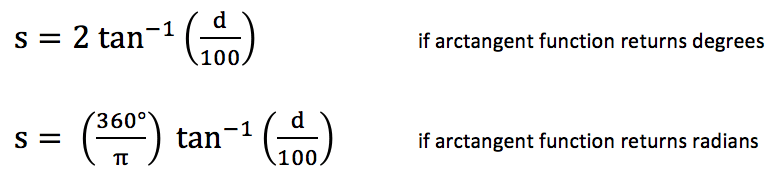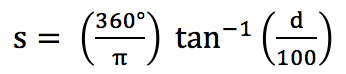# Two Places, Same Meteor?

A good friend of mine, Paul Martsching, records meteor activity many nights a year for the American Meteor Society near Ames, Iowa, and has been doing so for many years.  On some of those nights, I am also recording meteor activity near Dodgeville, Wisconsin.  Is it possible for both of us to see the same meteor?

Paul’s observing location near Ames and my observing location near Dodgeville are separated by 180 miles.  Meteors burn up in the atmosphere at an altitude of about 50 miles.  Using a little simple trigonometry, we can find that the parallax angle between where Paul and I see the meteor is about 122°.  So, a meteor at either of our zeniths would be below the horizon at the other location.  If, on the other hand, Paul saw a bright meteor 29° above his NE horizon, I might be able to see the same meteor 29° above my SW horizon.

In general, if two observers are separated by a distance d in miles, then they will see the location of the meteor in the sky shifted by approximately s°, as given in the following equation:This equation assumes that the curvature of the Earth is negligible, a reasonable assumption only when the two observers are relatively close to one another.

A more generalizable equation, taking into account the curvature of the Earth, though still assuming a spherical Earth is:Plugging in the numbers, we getWe essentially get the same answer—a parallax angle of 122°.  In fact, using the small angle approximation tan x ≅ x for x << 1 (where tan x is in radians), the equation above simplifies toIf this looks a little familiar, it is.  Assuming the meteor burns up at an altitude of 50 miles, the equation immediately above becomeswhich is our original equation!  So, for distances on the order of 200 miles or so (or less) we can completely ignore the curvature of the Earth.